CV算法恩仇录

V1

2022/08/19阅读：9主题：默认主题

# 初识人工智能原理

## 初识人工智能原理

• 人工智能数据的几何意义
• 通俗理解人工智能原理
• 理解一元线性回归与梯度下降的公式
• 通过房价预测案例理解多元线性回归
• 理解欠拟合和过拟合

### 人工智能数据的几何意义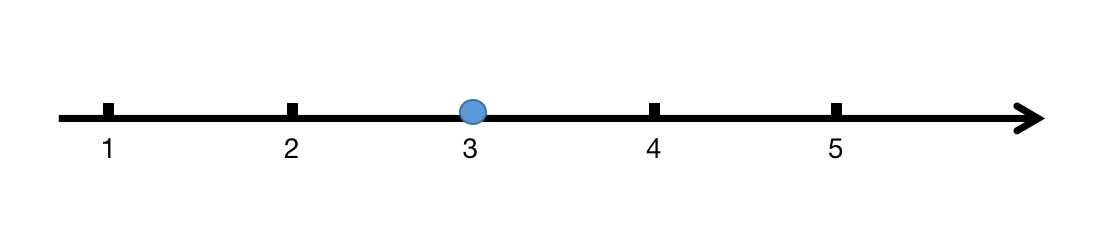图2： 一维空间的数值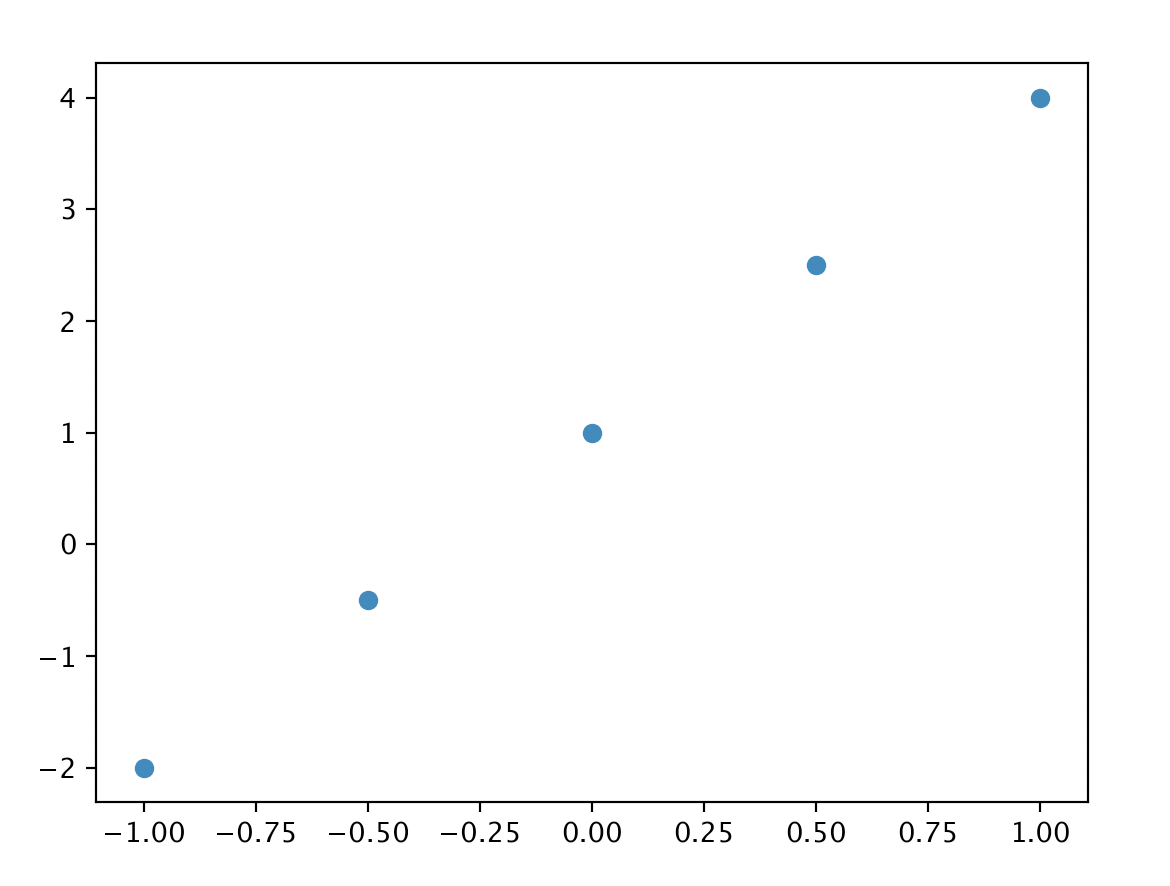图3： 二维空间的数据

### 通俗理解人工智能原理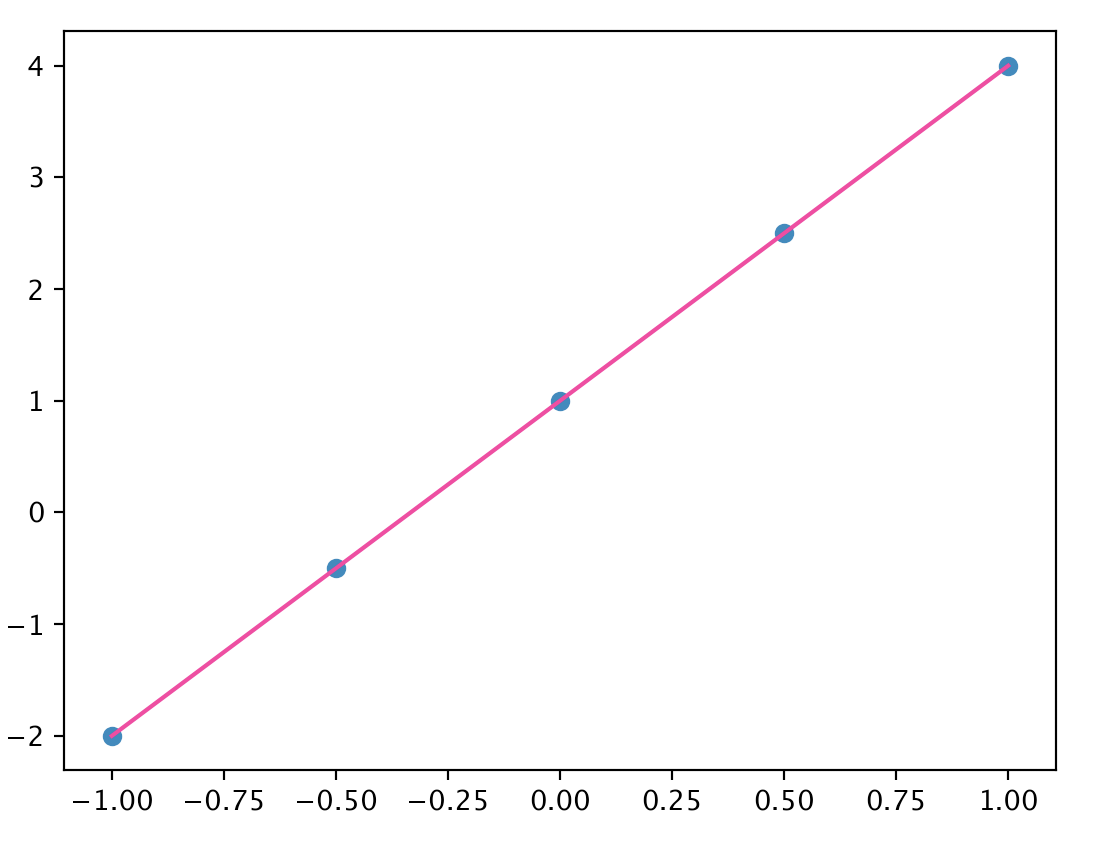图4： 直线

# 导入 numpy 库，对矩阵数据进行操作import numpy as npimport matplotlib.pyplot as plt%matplotlib inline# 将预设好的数据读取进来data_path = './data.npz'data = np.load(data_path)# 读取特征 xx = data['x']# 读取标签 yy = data['y']# 绘制散点图plt.scatter(x, y)plt.show()

'''定义线性函数   def () 的方式是定义一个函数'''def func(x, a, b):    return a * x + b# 给 a 和 b 一个初始值，都为1a = 1b = 1# 在区间 [1, 5] 内平均取 1000 个点x_line = np.linspace(1, 5, 1000)# 根据取的点套用公式得到直线y_line = func(x_line, a, b)# 绘制数据散点图，用作对比用plt.scatter(x, y, s=20, alpha=0.4, label="data")# 绘制直线plt.plot(x_line, y_line, lw=5, color="#990000", alpha=0.5, label="predict")plt.legend()plt.show()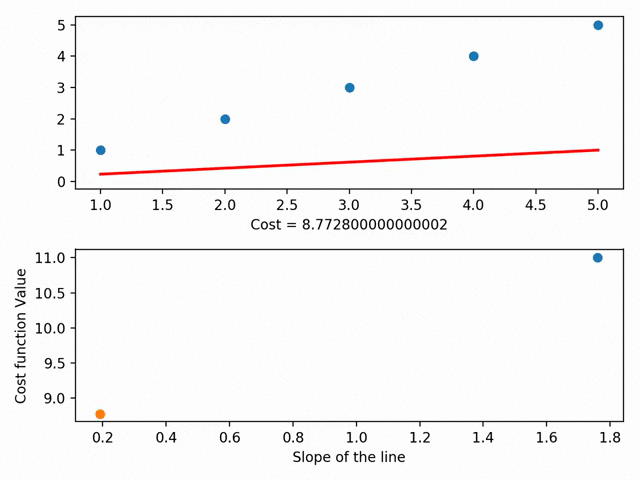图5： 不同数值的尝试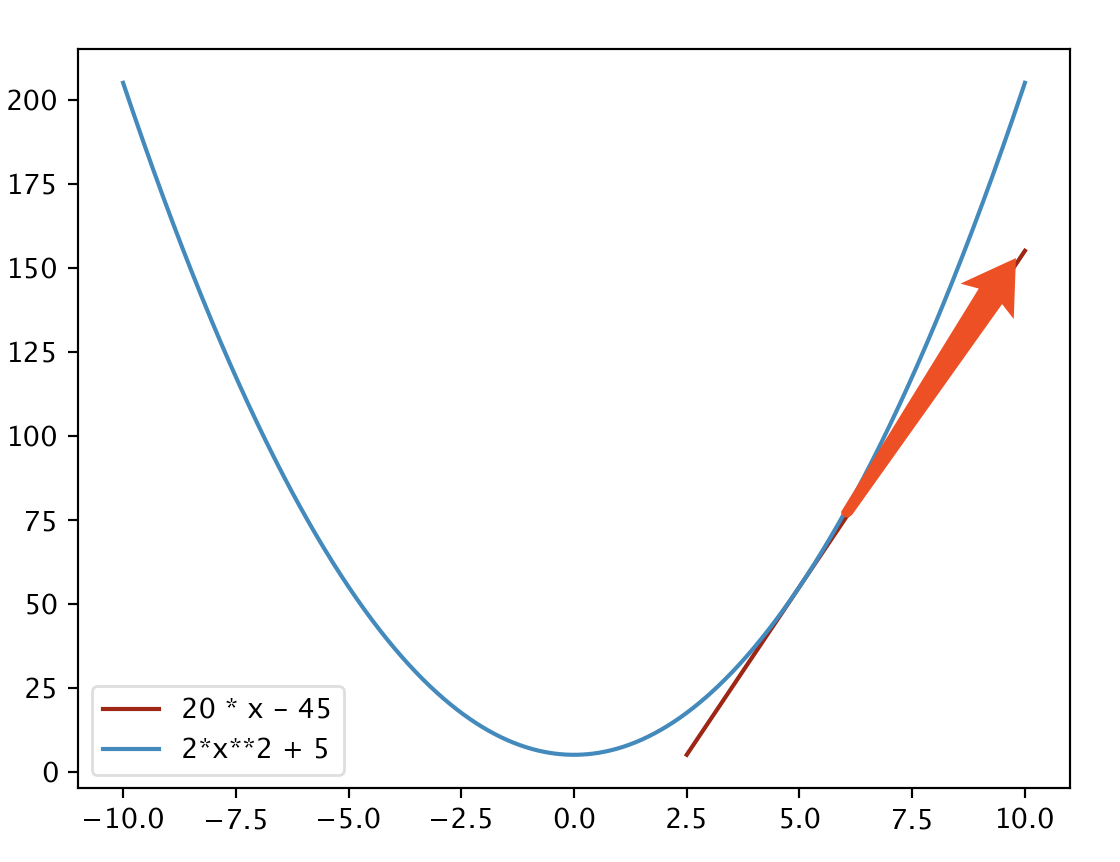图6： 导数

# 定义偏导数函数def grad_func(x, d, a, b):    '''    根据当前输入的x、d、a、b，求a和b的偏导    Arguments：        x:输入的x        d:真实值  label  y_label        a:当前a的值        b:当前b的值    '''    y = func(x, a, b)    # a的偏导数    grad_a = 2 * (y - d) * x    # b的偏导数    grad_b = 2 * (y - d)    return grad_a, grad_b

# 学习率eta1 = 0.05# 每轮学习数据量batch_size = 32# 进行1000次循环，也就是学习1000轮for itr in range(1000):    # 随机获取batch_size个数据的index    idxs = np.random.randint(0, len(x), batch_size)    # 取出随机的batch_size个数据    inx = x[idxs]    # 取出随机的batch_size个标签    ind = y[idxs]    # 计算grad_a与grad_b    ga, gb = grad_func(inx, ind, a, b)    # 求均值，除以batch_size    sum_ga = np.sum(ga) / batch_size    sum_gb = np.sum(gb) / batch_size    # 完成更新    a -= eta1 * sum_ga    b -= eta1 * sum_gbprint (a)print (b)# 再次绘制直线观察结果x_line1 = np.linspace(1, 5, 1000)# 根据取的点套用公式得到直线y_line1 = func(x_line1, a, b)# 绘制数据散点图，用作对比用plt.scatter(x, y, s=20, alpha=0.4, label="data")# 绘制直线plt.plot(x_line1, y_line1, lw=5, color="#990000", alpha=0.5, label="predict")plt.legend()plt.show()
5.190093956671917
-13.579019860865177V1